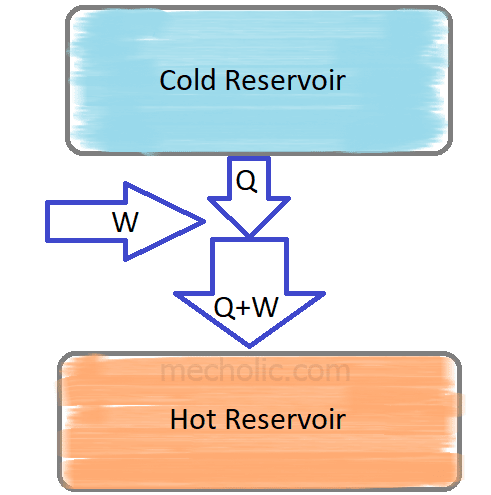Coefficient of Performance and Relative Coefficient of Performance of Refrigeration Cycle | Mecholic -->

# Coefficient of Performance and Relative Coefficient of Performance of Refrigeration Cycle## Coefficient of performance of refrigeration

The refrigerator is a device that extracts heat from colder part/ reservoir of the system and delivered it to the hotter sink/ environment. According to the second law of thermodynamics, the heat flow from a cold body to a hot body occur only by doing some outside work. The coefficient of performance of a refrigerator is defined as the ratio of useful cooling effect provided by the refrigerator to the work required.

Mathematically theoretical coefficient of performance of refrigeration is denoted as follows
Theoretical C.O.P. = Q/W
Where, Q = the total heat extracted in refrigeration (amount of cooling effect)
W = amount of work done by the refrigeration system

- What are the temperature limitations for refrigeration cycle (Reversed Carnot)?

## Relative coefficient of performance

A cooling system usually has heat loss during the operation. So its C.O.P is always slightly different from its theoretical C.O.P., the new C.O.P. is known as Actual C.O.P. Relative coefficient of performance defined as the ratio of actual C.O.P. to the theoretical C.O.P.

Relative C.O.P. = Actual C.O.P./Theoretical C.O.P.

- Comparison between COP of Heat Engine, Refrigerator, and Heat Pump
- Open and Closed Type of Refrigeration System – Advantages and Application